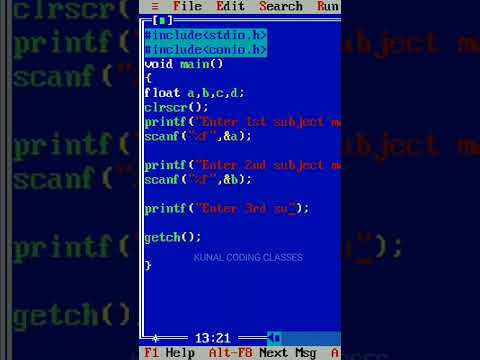# How to find percentage of 3 subject in c language

How to find percentage of 3 subject in c languageThis video contain proper explanation of how to find the %of marks of student exams if you have 4 subject then you have to make 5 float variables (LIKE float a,b,c,d,e;) and use this formula [ e=(a+b+c+d)/4]. and print output of e to get answers
if you have 5 subjects then you have to make 6 float variables and use formula [ f=(a+b+c+d+e)/5 ]